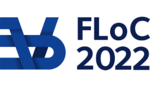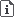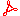FLOC 2022: FEDERATED LOGIC CONFERENCE 2022
PC ON SUNDAY, JULY 31ST
Days:
next day
all days

View: session overviewtalk overviewside by side with other conferences

08:30-09:00Coffee & Refreshments
10:30-11:00Coffee Break
12:30-14:00Lunch Break

Lunches will be held in Taub hall and in The Grand Water Research Institute.

14:00-15:30 Session 14K
Location: Ullmann 309
14:00
Towards higher order proof complexity
PRESENTER: Anupam Das

ABSTRACT. A nonuniform version of elementary computation is naturally given by 'higher-order' Boolean logic, an extension of propositional logic by abstraction and application operations at all finite types. The corresponding Frege-style proof system is nothing more than Church's simple type theory (STT), restricted to Boolean ground type. Our starting point is the observation that this system in fact bears correspondence with the well-known arithmetic theory IDelta0 + exp, a well-known theory of elementary computation¸ in the usual sense of proof complexity.

In this work-in-progress, we explore the gap between traditional bounded arithmetic theories in proof complexity (typically below exponential-time) and weak theories of arithmetic in proof theory (typically above elementary computation). The main idea is to formulate higher-order versions of Buss' classical theories that climb up the elementary hierarchy at the same time as analogous restrictions on cuts in STT. The goal is to establish modular characterisations for stages of the elementary hierarchy, and also intermediate alternating-time-hierarchies by way of (higher-type) quantifier complexity.

14:30

ABSTRACT. The CSP (constraint satisfaction problems) is a class of problems deciding whether there exists a homomorphism from an instance relational structure to a target one. The CSP dichotomy is a profound result recently proved by Zhuk and Bulatov. It says that for each fixed target structure CSP is either NP-complete or p-time. Zhuk's algorithm solves CSP in polynomial time for constraint languages having a weak near unanimity polymorphism.

For negative instances of p-time CSP it is reasonable to explore their proof complexity. We show that the soundness of Zhuk's algorithm can be proved in the theory of bounded arithmetic, namely in theory V^1 augmented by three special universal algebra axioms.

15:00
Proof complexity of natural formulas via communication argumentsPRESENTER: Dmitry Itsykson

ABSTRACT. A canonical communication problem $\mathrm{Search}{\phi}$ is defined for every unsatisfiable CNF $\phi$: an assignment to the variables of $\phi$ is distributed among the communicating parties, they are to find a clause of $\phi$ falsified by this assignment. Lower bounds on the randomized $k$-party communication complexity of $\mathrm{Search}{\phi}$ in the number-on-forehead (NOF) model imply tree-size lower bounds, rank lower bounds, and size-space tradeoffs for the formula $\phi$ in the semantic proof system $\mathrm{T}^{cc}(k,c)$ that operates with proof lines that can be computed by $k$-party randomized communication protocol using at most $c$ bits of communication [Göös, Pitassi, 2014]. All known lower bounds on $\mathrm{Search}{\phi}$ (e.g. [Beame, Pitassi, Segerlind, 2007]; [Göös, Pitassi, 2014], [Impagliazzo, Pitassi, Urquhart, 1994]) are realized on ad-hoc formulas $\phi$ (i.e. they were introduced specifically for these lower bounds). We introduce a new communication complexity approach that allows establishing proof complexity lower bounds for natural formulas.

First, we demonstrate our approach for two-party communication and apply it to the proof system $\mathrm{Res}(\oplus)$ that operates with disjunctions of linear equalities over $\mathbb{F}_2$ [Itsykson, Sokolov, 2014]. Let a formula $\mathrm{PM}_G$ encode that a graph $G$ has a perfect matching. If $G$ has an odd number of vertices, then $\mathrm{PM}_{G}$ has a tree-like $\mathrm{Res} oplus)$-refutation of a polynomial-size [Itsykson, Sokolov, 2014]. It was unknown whether this is the case for graphs with an even number of vertices. Using our approach we resolve this question and show a lower bound $2^{\Omega(n)}$ on size of tree-like $\mathrm{Res}(\oplus)$-refutations of $\mathrm{PM}_{K_{n+2,n}}$.

Then we apply our approach for $k$-party communication complexity in the NOF model and obtain a $\Omega\left(\frac{1}{k} 2^{n/2k - 3k/2}\right)$ lower bound on the randomized $k$-party communication complexity of $\mathrm{Search} mathrm{BPHP}^{M}_{2^n}}$ w.r.t. to some natural partition of the variables, where $\mathrm{BPHP}^{M}_{2^n}$ is the bit pigeonhole principle and $M=2^n+2^{n(1-1/k)}$. In particular, our result implies that the bit pigeonhole requires exponential tree-like $\mathrm{Th}(k)$ proofs, where $\mathrm{Th}(k)$ is the semantic proof system operating with polynomial inequalities of degree at most $k$ and $k= \O(\log^{1-\epsilon} n)$ for some $\epsilon > 0$. We also show that $\mathrm{BPHP}^{2^n+1}_{2^n}$ superpolynomially separates tree-like $\mathrm{Th}(\log^{1-\epsilon} m)$ from tree-like $\mathrm{Th}(\log m)$, where $m$ is the number of variables in the refuted formula.

The talk is based on the paper published in Proceedings of CCC-2021.

15:30-16:00Coffee Break
16:00-17:30 Session 19I
Location: Ullmann 309
16:00
Strong proof systems based on algebraic circuits

ABSTRACT. Grochow and Pitassi (2018) introduced IPS, the strongest algebraic proof system based on algebraic circuits. This is, however, not the only algebraic proof system of this sort: circuits can be restricted and can be represented differently. Additionally, similar proof systems based on inequalities can be considered.

In this talk I survey such systems and relations between them and announce recent developments.

17:00
On combinatorial principles coming from semi-algebraic proof systems